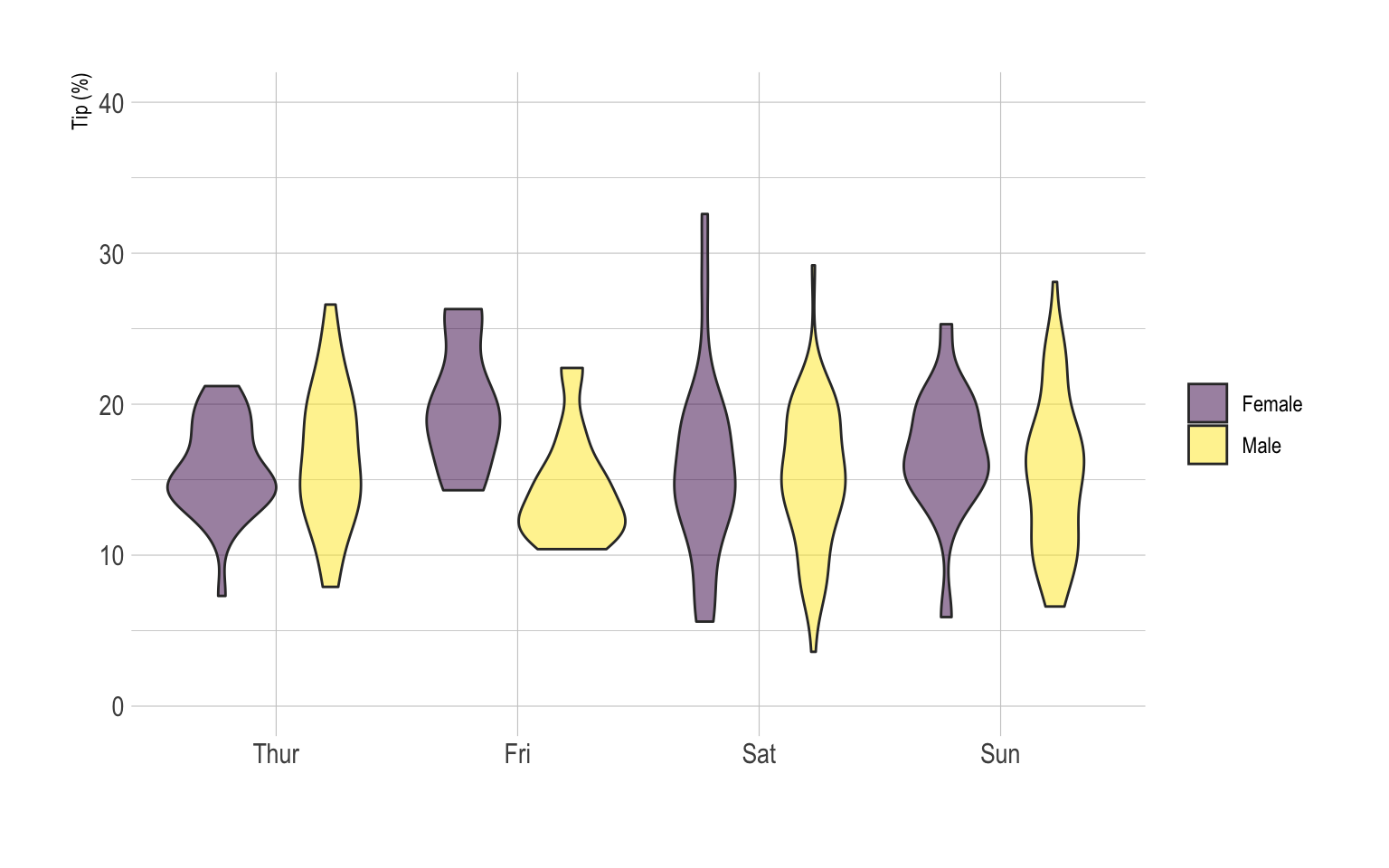# Grouped violin chart with ggplot2

This is an extension of the classic violin plot. Here, data are organized in groups and subgroups, allowing to build a grouped violin chart. Chart is implemented using `R` and the ggplot2 library.

A grouped violin plot displays the distribution of a numeric variable for groups and subgroups. Here, groups are days of the week, and subgroups are Males and Females. Ggplot2 allows this kind of representation thanks to the `position="dodge"` option of the `geom_violin()` function. Groups must be provided to `x`, subgroups must be provided to `fill`.

``````# Libraries
library(ggplot2)
library(dplyr)
library(forcats)
library(hrbrthemes)
library(viridis)

mutate(tip = round(tip/total_bill*100, 1))

# Grouped
data %>%
mutate(day = fct_reorder(day, tip)) %>%
mutate(day = factor(day, levels=c("Thur", "Fri", "Sat", "Sun"))) %>%
ggplot(aes(fill=sex, y=tip, x=day)) +
geom_violin(position="dodge", alpha=0.5, outlier.colour="transparent") +
scale_fill_viridis(discrete=T, name="") +
theme_ipsum()  +
xlab("") +
ylab("Tip (%)") +
ylim(0,40)``````Related chart types

## Contact

This document is a work by Yan Holtz. Any feedback is highly encouraged. You can fill an issue on Github, drop me a message on Twitter, or send an email pasting yan.holtz.data with gmail.com.